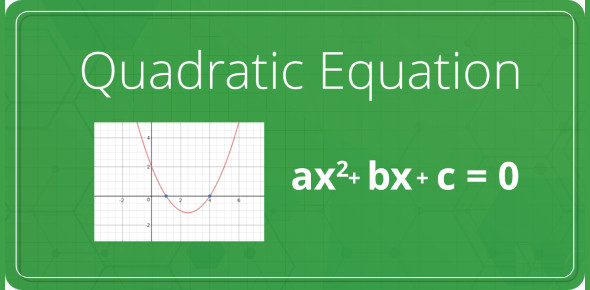# Quadratic Vocabulary Quiz: MCQ Test!

10 Questions | Attempts: 271
ShareSettingsQuadratic vocabulary quiz: MCQ test! Is math not that difficult? There are different ways that one can solve a quadratic expression, and the quiz below is designed to help see just how equipped you are to solve different problems. Do take up the quiz and get to see if you might need a refresher in solving quadratic equations. Keep an eye out for more quizzes just like it when you get done.

• 1.
What is an expression?
• A.

A formula with an equals sign.

• B.

A formula with a plus sign.

• C.

A formula without a plus sign.

• D.

A formula without an equals sign.

• 2.
Which best describes a quadratic equation?
• A.

An equation that has something raised to the second power.

• B.

An equation that has 2x as one of it's terms.

• C.

An equation that has no power that is greater than 2.

• D.

An equation where x is raised to the second power and that is the highest power on x.

• 3.
What is a polynomial?
• A.

An expression or equation with more that one term.

• B.

An expression or equation with one term.

• C.

An expression or equation with x raised to the second power and no higher power on x.

• D.

An expression or equation with three terms.

• 4.
The roots of a quadratic function tell you what about the function?
• A.

What the highest power is.

• B.

Where the graph of the function crosses the y-axis.

• C.

Where the graph of the function crosses the x-axis.

• D.

How many times the graph changes direction.

• 5.
A trinomial has _________ terms in it.
• 6.
Another word for the zero's of a quadratic functions is ___________.
• 7.
What is the vertex of a quadratic function?
• A.

The Maximum point of the quadratic.

• B.

The Minimum point of the quadratic.

• C.

The point that if you folded it, it would match up perfectly.

• D.

A and B.

• E.

None of these.

• 8.
What is the axis of symmetry?
• A.

￼The Maximum point of the quadratic.

• B.

The Minimum point of the quadratic.

• C.

The point that if you folded it, it would match up perfectly.

• D.

A and B.

• E.

None of these.

• 9.
The standard form of a quadratic is like _____________
• 10.
The greatest common factor in an expression is the __________ thing that divides into every term.

## Related TopicsBack to top
×

Wait!
Here's an interesting quiz for you.